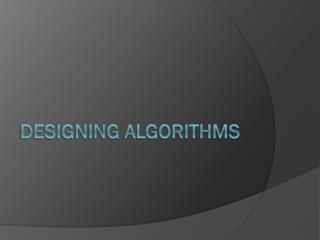# Designing Algorithms - PowerPoint PPT PresentationDownload PresentationDesigning Algorithms

Designing AlgorithmsDownload Presentation## Designing Algorithms

- - - - - - - - - - - - - - - - - - - - - - - - - - - E N D - - - - - - - - - - - - - - - - - - - - - - - - - - -
##### Presentation Transcript

1. Designing Algorithms

2. Art or Science? • Up for Debate • Creative Process • Decision after Decision – any of which can be a mistake. • What thought process can we follow?

3. Questions to Ask • Do I really understand the problem? • What does the input consist of? • What are the desired results of the program? • Can I construct an input example small enough to solve by hand? What happens when I try to solve it? • How important is it to my application that I always find the optimal answer? Can I settle for something close? • How large is a typical instance of my problem? 10 items? 1,000? 1,000,000? • How important is speed? Solved in 1 second, 1 hour, 1 day? • How much time and effort can I invest in implementation? Simple algorithms that take a day or more complex ones that take longer to code correctly? • What category of problem am I trying to solve? Numerical? Graph? Geometric? Strings? Sets? Which formulation seems easiest?

4. Questions to Ask • Can I find a simple algorithm or heuristic for my problem? • Will brute force correctly solve the problem? • If so, how am I sure it is always correct? • How do I measure the quality of the solution? • Does it run in polynomial or exponential time? Is that ok for this problem? • Is the problem defined well enough to have a correct solution? • Can I solve my problem repeatedly by trying some simple rule, like choosing the largest, smallest, or a random item first? • If so, on what inputs does this work well? Are these inputs expected for this problem? • On what inputs does this work poorly? If none, can I show it always works well? • How fast do I get an answer? Is the implementation simple?

5. Questions to Ask • Is my problem in the catalog of algorithmic problems in the back of this book or another algorithms book? • What is known about the problem? Is there already an implementation available I can use? • Did I look in the right place for my problem? Look at the index, pictures, etc? • Are there relevant resources on the WWW? What does Google find? How about the book’s website? (http://www.algorist.com/)

6. Questions to Ask • Are there special cases of the problem I know how to solve? • Can it be solved efficiently if some parameters are ignored? • Is it easier to solve with trivial input values (like 0 or 1)? • Can I simplify the problem to the point where I can solve it efficiently? • Why can’t this special-case algorithm be generalized to a wider class of inputs? • Is my problem a special case of a more general problem that has already been solved?

7. Questions to Ask • Which of the standard algorithm design paradigms are most relevant to my problem? • Sets to be sorted? • Divide and Conquer? • Dynamic Programming? • Use of particular data structures? • Random Sampling? • Linear programming? • Is it NP-Complete?

8. Questions to Ask • Am I still stumped? • Willing to spend money to bring in an expert (unfortunately not an option until after you graduate) • Go back to the beginning and ask the questions again!

9. Problem Solving • Part art, part skill. Well worth developing!